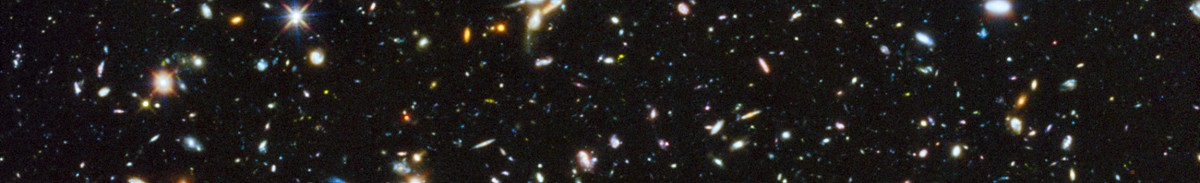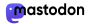Original image by NASA, ESA, H. Teplitz and M. Rafelski (IPAC/Caltech), A. Koekemoer (STScI), R. Windhorst (Arizona State University), and Z. Levay (STScI); cropped by L. Marmet.

# Cosmology Calculators

Recent observations by the Webb Telescope (JWST) have shown a large number of galaxies at high redshifts.  Contrary to expectations based on galaxy-formation models and the Standard Model of Cosmology (ΛCDM), these galaxies appear small and very bright.

The Hubble constant derived from the expansion rate of the local universe is in tension with the value derived from the Cosmic Microwave Background Radiation (CMBR) released by the hot Big Bang.  This "Hubble tension" is forcing cosmologists to question every aspect of the ΛCDM model.

This webpage hosts two Cosmology Calculators: one for the ΛCDM model, the other for a Static Cosmology with Stimulated-Transfer redshift (SSTz).

Enter values and the results will appear immediately.

## ΛCDM Cosmology

Based on the calculator written by Ned Wright (2006, PASP, 118, 1711):
http://www.astro.ucla.edu/~wright/CosmoCalc.html.  The calculation includes three massless neutrino species and a correction for annihilations of particles not present now (e.g. e+/e ).

The Hubble parameter is  H2(z) = H02 (ΩM(1 + z)3 + Ωk(1 + z)2 + ΩΛ).

The size evolution of star-forming galaxies follows the power law

re = r0 (1 + z)nS .

The Tolman test for expansion predicts a surface brightness  <SB>  that decreases as  (1 + z)4. Luminosity evolution produces the Tolman signal

Δ<SB>evol = 2.5 log (1 + z)4-nL mag,
ΔMevol = 2.5 log (1 + z)nL mag.

These relations are used for the calculations of reported observations, without model-dependent evolution corrections.

## SSTz Cosmology

The distance-redshift relation in a Static Cosmology is obtained from the photon energy loss per unit distance  d(hν)/dr = -Hz(ne) hν, where Hz(ne) is proportional to the number density of free electrons.  Ponderomotive forces produce a Stimulated-Transfer redshift that is independent of wavelength, with the distance-redshift relation

dA = (1/Hz) ln(1 + z).

In a flat and static cosmology, the radial distance  r  is equal to the angular distance dA.   The photon energy being proportional to (1 + z)-1 implies a bolometric luminosity distance

dL = (1 + z)1/2 dA,

with an additional (1 + z)1/2 factor for the redshift of a transient event that conserves the number of photons (e.g. supernovae light-curves).

A distant object at temperature T is seen by an observer as a blackbody at the Wien temperature Tw(z) = T/(1 + z), but with a spectral radiance enhanced by a factor of (1 + z)3,

Bν(ν, Tw, z) = (1 + z)3  (2hν3/c2) [ehν/(kTw) - 1]-1.

## Parameters

km/s/Mpc

Open: uses  ΩΛ = 0  giving an open Universe [if you entered ΩM < 1].
Flat:  uses  ΩΛ = 1 - ΩM  giving a flat Universe.
General: uses the ΩΛ that you entered.

used for sample selection
introduces a bias, c.f. 'Fundamental Plane' scaling relations.
/Gpc

Euclidean
(Light-matter interactions provide the pressure
that counterbalances gravitation.)

## ΛCDM Cosmology

*** JavaScript not enabled or script file not updated ***

Source for default parameters H0, ΩM and ΩΛ
Source for default parameter nS
Source for default parameter nL
Source for default parameter nB

## SSTz Cosmology

*** JavaScript not enabled or script file not updated ***

Source for default parameter Hz
all parameters to default values.
which H0 gives reported dA(ΛCDM) = dA(SSTz).

## Discussion

It is difficult to compare the two models to a high accuracy. Astrophysical data are analyzed with the assumptions of ΛCDM cosmology and corrected for various effects in powers of 1 + z (e.g. luminous flux, time dilation, galactic evolution, Hubble residuals, deceleration parameter, dust reddening, etc.)

Both calculators give observed quantities as interpreted in the framework of the models. Other effects are difficult to disentangle from an analysis based on the ΛCDM model.

Interpreting observations with the ΛCDM model one concludes that: "compared to typical galaxies at later times, [young galaxies in the first billion years of cosmic time] show more extreme emission-line properties, higher star formation rates, lower masses, and smaller sizes." [Ref. (2017)]  This is confirmed by new observations from the Webb Telescope.  In a static cosmology, galaxies are farther away and their stars appear to have a blackbody spectrum with an excess brightness.

Louis Marmet
@redshiftdrift@astrodon.social onLast update: 2023-4-24

https://www.websiteout.net/counter.php